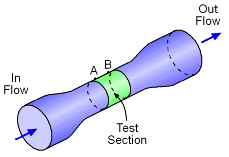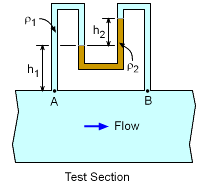Ch 2. Fluid Statics Multimedia Engineering Fluids PressureVariation PressureMeasurement HydrostaticForce(Plane) HydrostaticForce(Curved) Buoyancy
 Chapter 1. Basics 2. Fluid Statics 3. Kinematics 4. Laws (Integral) 5. Laws (Diff.) 6. Modeling/Similitude 7. Inviscid 8. Viscous 9. External Flow 10. Open-Channel Appendix Basic Math Units Basic Equations Water/Air Tables Sections Search eBooks Dynamics Fluids Math Mechanics Statics Thermodynamics Author(s): Chean Chin Ngo Kurt Gramoll ©Kurt GramollFLUID MECHANICS - CASE STUDY IntroductionWind Tunnel Experiment A wind-tunnel experiment is conducted to study the effect of wind on tall buildings. In order to obtain the volumetric flow rate through the test section of the wind tunnel, engineers are first asked to determine the pressure drop between points A and B using a differential U-tube manometer. The fluid in the wind tunnel has a density of 1000 kg/m3 while the density of the gage fluid is 1600-kg/m3. Column heights are given as h1 = 1.25 m and h2 = 0.75 m.U-Tube Differential Manometer Question First derive an equation for the pressure drop (pA - pB). Then, calculate the value of (pA - pB) for the given values of fluid densities and column heights. Approach Although the fluid in the wind tunnel is flowing, the fluid in the manometer remains stationary. Hence, hydrostatic pressure analysis is still valid to determine the pressure drop.

Practice Homework and Test problems now available in the 'Eng Fluids' mobile app
Includes over 250 problems with complete detailed solutions.
Available now at the Google Play Store and Apple App Store.